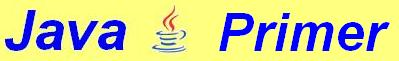3. Variables and repetition

Suppose we want to draw six concentric circles (circles with the same centre), we could draw the separate circles like this:

g.drawOval (50, 10, 220, 220);
g.drawOval (70, 30, 180, 180);
g.drawOval (90, 50, 140, 140);
g.drawOval (110, 70, 100, 100);
g.drawOval (130, 90, 60, 60);
g.drawOval (150, 110, 20, 20);

But a better idea is to use a repetition structure, like this:

// Six circles drawn with a do/while loop
import java.applet.*;
import java.awt.*;
class Circles2 extends Applet {

public void paint (Graphics g) {

int radius = 110, width = 320, height = 240;
do {

}
}

The program sets out drawing a circle with a radius of 110 pixels. Repeatedly 20 is subtracted, and every time a circle is drawn with this new radius -- as long as the radius is greater than zero. This kind of repetition can be accomplished in Java with:
do {
// The text is indented here
// to indicate that this part depends
// on the do/while construction.
A remarkable expression is this one:
You can also write it in a different way:
In either case the meaning is that 20 is subtracted from the radius.

Drawing lines
Any point in the applet window can be described by coordinates. For instance, in the applet shown here you see the point (200, 100), which means that it lies 200 pixels from the left and 100 pixels from the top.

In Java you can draw a line from point (x1, y1) to (x2, y2) with:

g.drawLine (x1, y1, x2, y2);
You can subdivide the applet window into horizontale strips of 10 pixels high with:
// The window is divided into horizontal strips.
import java.applet.*;
import java.awt.*;
public class HorizontalLines extends Applet {
public void paint (Graphics g) {
int y = 10, width = 320, height = 240;
do {
g.drawLine (0, y, width, y);
y += 10;
} while (y < height);
}
}
This gives us the following image:

 Exercise 3.1 Write an applet that divides the window into squares of 10 pixels wide and 10 pixels high. Exercise 3.2 Write an applet in which lines are drawn at 10 pixel intervals from the bottom left-hand corner to the top right-hand corner, and so on from the top left-hand corner to the bottom right-hand corner. It may be useful to know that <= means"smaller than or equal to".

(c) Thomas J.H. Luif, 2003